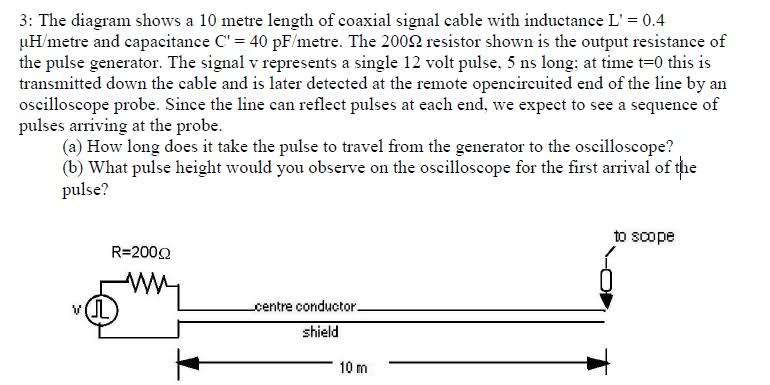# Pulse Through Cable Help Please!

mmmboh
Hi, this is the question:For part a) I did V=(L/C)-1/2=2.5x108 m/s. And then I said T=x/v where x=10m, so T=4x10-8s.

For part b) V++V-=VL...since it's the first wave I suppose there wouldn't be a reflecting wave yet, so V-=0.
I have an equation that says V+=[VL/2](1+R0/RL)...since it is an open circuit, RL=infinity, so R0/RL=0, and the equation becomes V+=VL/2 and VL=12V, so V+=pulse height = 6V.....but if $$V_-=0$$ then according to my first equation $$V_+=V_L=12V$$..I'm confused.

I have a feeling b) is wrong and that it should actually be 24V because of a combination of waves, I'm not sure about part a), any help please?

Mentor
Hi, this is the question:For part a) I did V=(L/C)-1/2=2.5x108 m/s. And then I said T=x/v where x=10m, so T=4x10-8s.

For part b) V++V-=VL...since it's the first wave I suppose there wouldn't be a reflecting wave yet, so V-=0.
I have an equation that says V+=[VL/2](1+R0/RL)...since it is an open circuit, RL=infinity, so R0/RL=0, and the equation becomes V+=VL/2 and VL=12V, so V+=pulse height = 6V.....but if $$V_-=0$$ then according to my first equation $$V_+=V_L=12V$$..I'm confused.

I have a feeling b) is wrong and that it should actually be 24V because of a combination of waves, I'm not sure about part a), any help please?

It looks like you used the equation for characteristic impedance:

http://en.wikipedia.org/wiki/Characteristic_impedance

instead of for the propagation velocity (of a lossless TL):

http://en.wikipedia.org/wiki/Wave_propagation_speed

And to your question about the amplitude at the first reflection, remember that you are driving the TL through the 200 Ohm resistor. That will play off against the Zo of the TL. And I believe they want you to include the reflection effects in calculating the initial open circuit voltage at the open end...

mmmboh
For the propagation velocity thing, is that for part a)? I used the same equation except without the c in the denominator because I don't need what fraction of the speed of light it is.

For part b), I'm not sure I understand what to do in that case, is the equation I used wrong?

Mentor
For the propagation velocity thing, is that for part a)? I used the same equation except without the c in the denominator because I don't need what fraction of the speed of light it is.

You posted for part a:

For part a) I did V=(L/C)-1/2

That's Zo.

mmmboh
Ooh right, I meant to put v=(LC)^-1/2 actually, that's the calculation I did, so the answer remains the same for part a).

mmmboh
Ok so from my equation for reflection coefficient, V-/V+(=RL-R0)/(RL+R0)...since there is an open circuit at the oscilloscope RL=infinity, and so V-/V+=1...so V- and V+ add together to make the pulse height at the oscilloscope 24 V......the answer is either that, or since Z0=100 ohm and the resistance in the generator is 200 ohm then V+=12V(100/300)=4V, unless it's 12V(200/300)=8V and then the V+ and V- still add and make 8V (or 16V depending on which equation is right) at the oscilloscope...

I think one of these two is the answer, maybe not, can you clear things up please?

Mentor
Ok so from my equation for reflection coefficient, V-/V+(=RL-R0)/(RL+R0)...since there is an open circuit at the oscilloscope RL=infinity, and so V-/V+=1...so V- and V+ add together to make the pulse height at the oscilloscope 24 V......the answer is either that, or since Z0=100 ohm and the resistance in the generator is 200 ohm then V+=12V(100/300)=4V, unless it's 12V(200/300)=8V and then the V+ and V- still add and make 8V (or 16V depending on which equation is right) at the oscilloscope...

I think one of these two is the answer, maybe not, can you clear things up please?

I believe your 2nd answer is correct. As long as the TL is electrically long enough so that you can't treat it as just a lumped capacitance, then you will get the 3:1 voltage division, and the full positive reflection at the open end should give you double the incident amplitude. At least until things start ringing all over the place from the multiple reflections.

mmmboh
Ok thanks a lot!